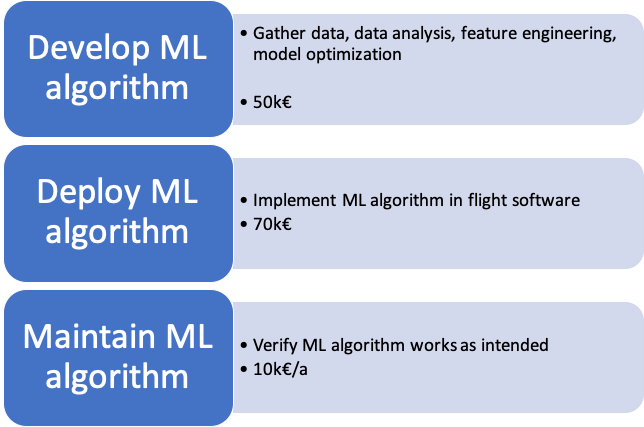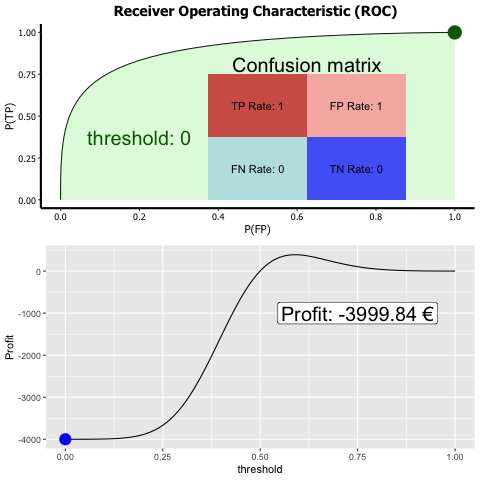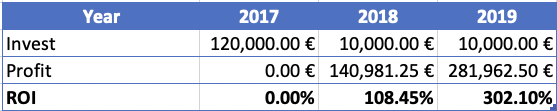## 24.10 Calculation of return of invest (ROI)

To calculate the ROI we need to calculate the profit as well as the invest. The following numbers are, for obvious reasons, not the real numbers.

Calculation of ROI:

• Calculate invest
• Calculate profit
• $$ROI = \frac{Profit}{Invest}$$

### 24.10.1 Calculation of ML project invest

To calculate the project invest the different phases of the ML project have to be considered

Phases of ML project:

• Development
• Deployment
• Maintenance

Estimating the invest is a bit tricky for ML projects because often there is not the same amount of heritage as for other software projects. Also the amount of effort to make the data available and accessible is difficult to estimate early in the project. As a rule of thumb the effort to gather the data and pre-process is about 80% of the total effort to develop a ML model.

Estimate development cost:

• Due to low heritage vague
• Cost related with data
• making them available
• making them accessible (e.g. data might be hand written)
• necessary data might only become clear during project
• Data handling could be 80% of development effort
• accessing data
• pre-processing data

The deployment of a ML algorithm can be simple but might also be an elaborated effort. In the example here the deployment costs are effort is considerable because software which runs on a satellite has to fulfill special requirements.

Deployment might happen in different stages, it might be deployed only for certain regions a certain kinds of customers. There might be the need for a feedback mechanism to improve the algorithm over time. Also the implementation might be such that it is easily scalable in case the use of the model increases potentially within a short time.

Estimate deployment cost:

• Deployment might happen in different stages
• regional
• certain groups of customer
• Might need feedback mechanism
• to improve algorithm
• to report issues
• fault
• bias
• unwanted behavior
• Scalability
• in case of demand increase
• increase might be sudden

Maintaining the ML algorithm shall ensure that the algorithm’s performance does not deteriorate over time and might even be increased, also that the algorithm runs safely and works in a maybe changing IT environment.

Estimate maintenance cost:

• Ensure safety
• IT environment might change
• new operating system version
• Ensure performance
• maybe include feedback
• could involve human intervention

Below the numbers for the previously described project are given. Please note that for confidential reasons the figures are not the real figures, but are in the right ballpark.The numbers are not the exact numbers of the project, but are in the right ballpark

### 24.10.2 Calculation of ML profit

For a given ROC the highest available profit can be calculated by assigning a price tag to the elements of the confusion matrix. The profit calculated is per day.

Calculation of ML profit:

• Assign price tag to elements of confusion matrix
• e.g. $$Profit=TP Rate *1000€ - FP Rate *5000€)$$
• Determine maximum profit per dayDummy profit numbers, exemplary graph

Choosing the probability threshold for maximum profit yields to a profit of 386.25€/d.

### 24.10.3 Resulting ROI

The resulting ROI is given for the years 2017-2019 in the following table:Dummy numbers, exemplary graph

The analysis shows that the ML algorithm not only improves the technical capability of the system but also would have an impressive ROI.